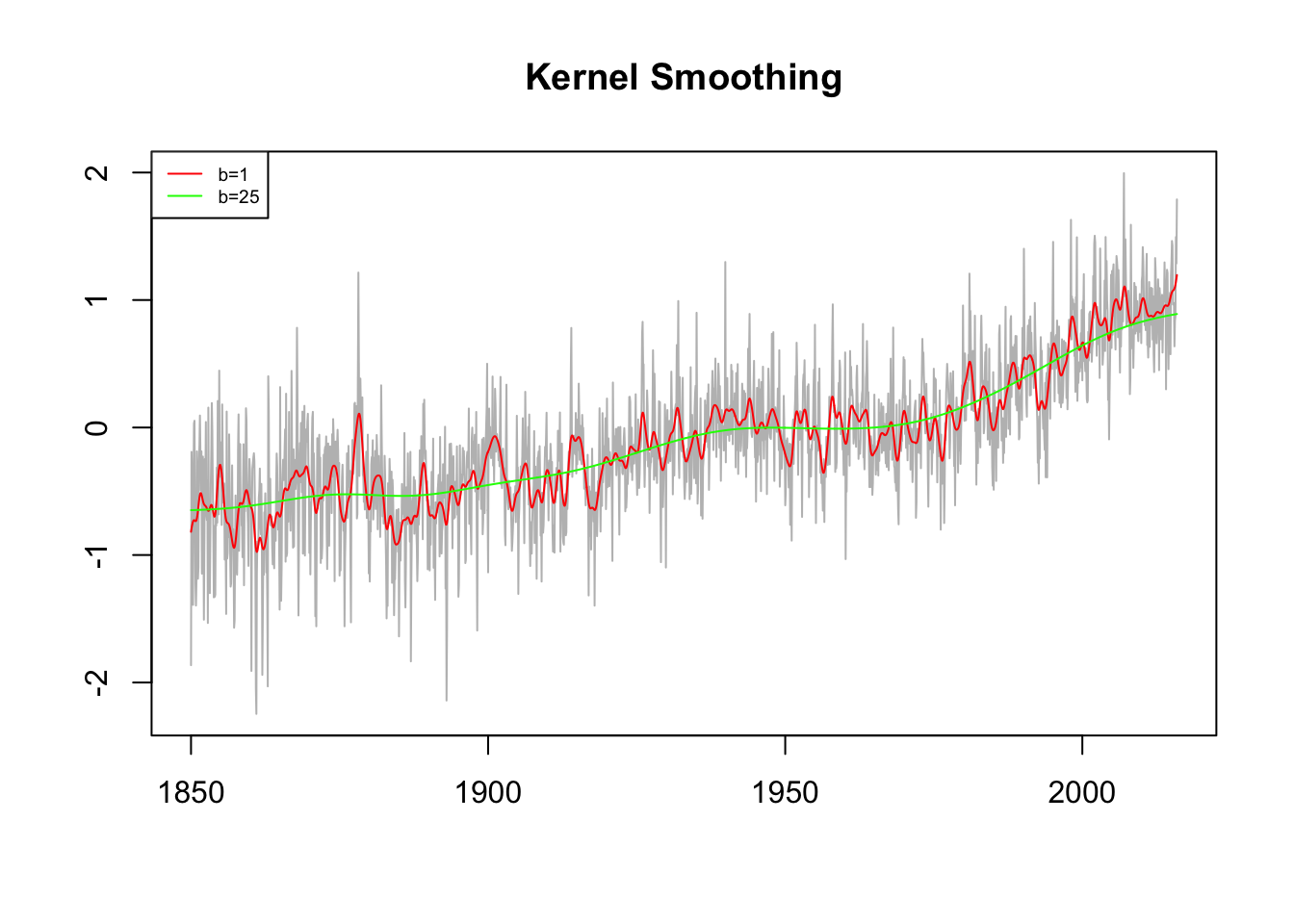Kernel smoothing is a moving average smoother that uses a weight function, also referred to as kernel, to average the observations. The kernel smoothing function is estimated by

$\hat f_t = \sum_{i=1}^b w_i(t)x_i\text{,}$

where

$w_i(t) = \frac{K \left(\frac{t-i}{b}\right)}{\sum_{i=1}^bK\left(\frac{t-i}{b}\right)}$

are the weights and $$K(\cdot)$$ is a kernel function, typically the normal kernel, $$K(z) = \frac{1}{\sqrt{2 \pi}}\exp(-z^2/2)$$. The wider the bandwidth, $$b$$, the smoother the result. In R we apply the ksmooth() function for kernel smoothing.

library(xts)

dt <- index(temp.global)
y <- coredata(temp.global)
plot(dt, y, type = 'l',
col = 'gray', xlab = "", ylab = "",
main = 'Kernel Smoothing')

lines(ksmooth(dt, y, "normal", bandwidth = 1),
col = 'red', type = 'l')
lines(ksmooth(dt, y, "normal", bandwidth = 25),
col = 'green', type = 'l')

legend('topleft',
legend = c('b=1','b=25'),
col = c('red', 'green'),
lty = 1,
cex = 0.6)